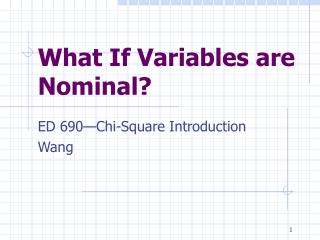DownloadDownload PresentationWhat If Variables are Nominal?

# What If Variables are Nominal?

Télécharger la présentation## What If Variables are Nominal?

- - - - - - - - - - - - - - - - - - - - - - - - - - - E N D - - - - - - - - - - - - - - - - - - - - - - - - - - -
##### Presentation Transcript

1. What If Variables are Nominal? ED 690—Chi-Square Introduction Wang

2. Types of Statistical Tests When running a t test and ANOVA • We compare: • Mean differences between groups • We assume • random sampling • the groups are homogeneous • distribution is normal • samples are large enough to represent population (>30) • DV Data: represented on an interval or ratio scale • These are Parametric tests!

3. Types of Tests When the assumptions are violated: • Subjects were not randomly sampled • DV Data: • Ordinal (ranked) • Nominal (categorized: brands of candy, types of car, levels of education, learning styles, Likert Scale) • The scores are greatly skewed or we have no knowledge of the distribution We use tests that are equivalent to t test and ANOVA Non-Parametric Test!

4. Chi Square • used when data are nominal (both IV and DV) • Comparing frequencies of distributions occurring in different categories or groups • Tests whether group distributions are different • Shoppers’ preference for the taste of 3 brands of candy • determines the association between IV and DV by counting the frequencies of distribution • Gender relative to study preference (alone or in group)

5. Types of Chi Square • A one-dimensional Chi Square (p. 503 table--Gay) • Determine if the observed frequencies are significantly different from the expected frequencies X Y Z (3 brands of candy) 40 30 20 (90 shoppers) • Null hypothesis: there is no difference between graduate students’ study preference

6. Types of CHI-SQUARE • A two-dimensional Chi Square (p. 504 table) • Frequencies are categorized along more than one dimensions • Gender relative to study preferences • Gender relative to voting for school voucher

7. Chi Square (by formula) • Hypothesis: Ho • Level of significance • Testing frequencies of categories • Computation of Chi square value • Determination of critical value • DF: R-1 (R is the no. of the categories--DV) • Check in Chi-square table • Obtained value > critical value • Decision: • Reject null hypothesis

8. Chi Square (by software) • Use Sample data • Culture-Color data; sales data • Answer the questions: • Differences: culture-color; region-product color • Follow the Chi-square handout • If p value < 0.05 • Decision: • Reject null hypothesis